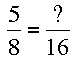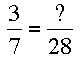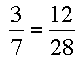SEARCH HOMEMath Central Quandaries & QueriesQuestion from Raymond, a parent: 5/8=?/16 ?=?We have two responses for you.

Hi Raymond,

What you are working with here is what is called equivalent fractions, meaning the second fraction is just a multiple of the first.In order to solve the question, you need to first ask yourself what operation was done to the 8 to change it to 16.  Once you have this answer, you can then perform the same operation to the 5 to find the question mark.

For example, if I have the following question:I first ask myself, what operation was done to the 7 to change it to 28.  Obviously, 7 is multiplied by 4 to become 28.  Knowing this, we simply multiply 3 by 4, which gives us 12.  This is now replaced for the question mark.Sara

Hi Raymond,
In order to find a fraction equivalent (the same size) to the one you were given, you must perform the same operation to the numerator (top number) and denominator (bottom number). Try this example:

1/2=?/8

We can see that 2 must have been multiplied by 4 in order to get 8 (4*2=8) by dividing 8 by 2 (8/2=4). So we must multiply the numerator by the same number in order to have an equivalent fraction.

1/2 * 4/4 = 4/8

Because 4/4 is really 1, we aren't really changing the value, just the way we write it.
MelanieMath Central is supported by the University of Regina and The Pacific Institute for the Mathematical Sciences.